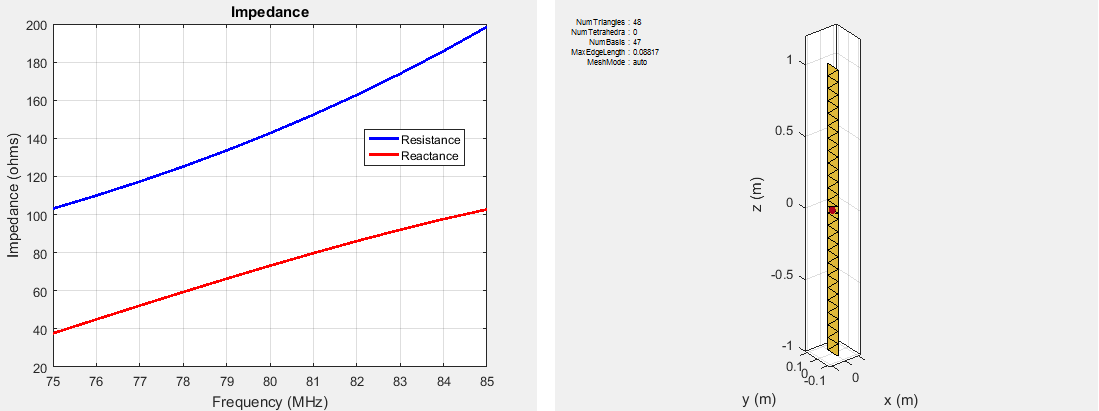## Meshing

Method of Moments (MoM) is a numerical method that transforms Maxwell’s continuous integral equations into an approximate discrete formulation that requires inversion of a large matrix. Meshing is the process of converting the continuous domain into the discrete domain for solving the equations. For discretizing surfaces, typically either triangles or rectangles are used. Antenna Toolbox™ uses triangular element for meshing as it conforms better for arbitrary shaped surfaces. The triangles are used to approximate the surface current using the Rao-Wilton-Glisson (RWG) basis functions. To get an accurate result, ensure that large number of triangles are present in the region where current variation is the highest. This region is typically either the corners in the antenna geometry or at the point where the antenna is excited.

### Automatic Meshing Mode

In Antenna Toolbox, the antenna structures mesh automatically based on the analysis frequency chosen. For analysis functions that accept a scalar frequency, the antennas mesh at that single frequency to satisfy the minimum triangles required. Then the functions calculate the corresponding antenna parameter.

```d = dipole; impedance(d,75e6); mesh(d)```In above example, the dipole is meshed at 75 MHz automatically before calculating the impedance at that value. Use the mesh command to view the meshed dipole. The number of triangles is 44.

For analysis functions that accept a frequency vector (`impedance`, `sparameters`, `returnLoss`, `vswr`), each antenna meshes once at the highest frequency. Then, the functions calculate the corresponding antenna parameters at all the frequencies in the range.

```d = dipole; impedance(d,75e6:1e6:85e6); mesh(d)```In above example, the dipole is meshed at the highest frequency, 85 MHz automatically before calculating the impedance at all the frequencies from 75 to 85 MHz. Meshing at the highest frequency, 85 MHz, ensures maximum number of triangles and a smoother plot of the dipole impedance. Use the mesh command to view the meshed dipole. The number of triangles is 48, which is more than single frequency meshing.

### Manual Meshing Mode

You can choose to mesh the structure manually at the highest frequency of interest. Manual meshing is done by specifying the maximum edge length that is used for discretizing the structure. One option is to specify the value to be one-tenth of the wavelength at the highest frequency of interest. For example:

```sp = spiralArchimedean; freq = 0.8e9:100e6:2.5e9; lambda = 3e8/freq(end); mesh (sp, MaxEdgeLength=lambda/10); ```

Alternatively, you can run an analysis at the highest frequency of interest and get the maximum edge length. Specify this maximum edge length using the `mesh` function as shown. This mesh is used for all other calculations.

```sp = spiralArchimedean; freq = 0.8e9:100e6:2.5e9; temp = axialRatio(sp,freq(end), 0, 90); meshdata = mesh(sp); mesh(sp, MaxEdgeLength=meshdata.MaxEdgeLength); ```

### Strip Meshing

For strip meshing, include at least 10 triangles per wavelength in a strip. This rule applies for structures such as dipoles, monopoles, and loops. Antenna Toolbox antenna meets the requirement automatically, based on the analysis frequency specified. The structured mesh generated in such cases is shown:### Surface Meshing

For surface meshing, it is recommended that there be at least 100 elements per wavelength in a particular area. This rule applies to structures such as spirals, patches, and ground planes in general. Antenna Toolbox antenna meets the requirement automatically, based on the analysis frequency specified. In these cases, a non-uniform mesh is generated as shown:Larger number of triangles are added in regions with higher current density.

### Meshing a Dielectric Substrate

For antennas using dielectrics and metals, Antenna Toolbox uses tetrahedrons to discretize the volume of the dielectric substrate.Thickness of the dielectric substrate is measured with respect to the wavelength. A dielectric substrate with thickness less than or equal to 1/50th of the wavelength is a thin substrate. When you mesh an antenna using dielectric in auto mode, thin substrates yield more accurate solutions.

A substrate with a thickness of 1/10th of the wavelength is a thick dielectric substrate. The method of moments solver requires 10 elements per wavelength to yield an accurate solution. Manual meshing yields more accurate solutions for antennas using thick dielectric substrate, as it satisfies the 10 elements per wavelength criteria.

 Makarov, S.N. Antenna and EM Modeling with MATLAB, New York: Wiley & Sons, 2002